# Sample Question: HMO Theory for 1,3-Butadiene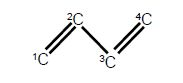(a) Write down the Hiickel secular determinant for the four C-atoms of butadiene in terms of x, where x = (α – E)/β.

(b) Solve the secular determinant for x and then rewrite the solutions in terms of (α – E)/ β and so find the four allowed quantized energies (eigenvalues).

(c) Evaluate the normalized wavefunction (eigenvector) for each energy (eigenvalues).

(d) Which of the wavefunctions is the HOMO or LUMO?

Solution

The solution of the Hfickel determinant for butadiene is shown below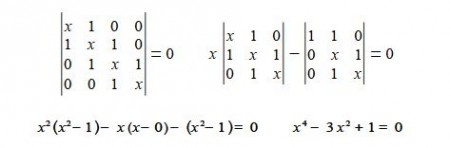To solve this quartic equation let us substitute y = x2 and then use the quadratic equation to solve for y (using an excess of significant figures to reduce rounding errors) we have,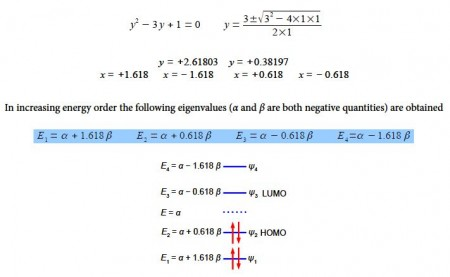Although solving this particular 4×4 secular determinant gives a quartic equation which may be solved manually to find the eigenvalues, as we have done above, for larger matrices this is too cumbersome. Also carrying out the matrix multiplication tp find the eigenvectors is less easy even for a 4×4 matrix. Luckily we can generalize the results that we already have which gives us a method that is a distant relative of the particle in a box quantized systems.

For an acyclic conjugated hydrocarbon containing n carbon atoms in the chain,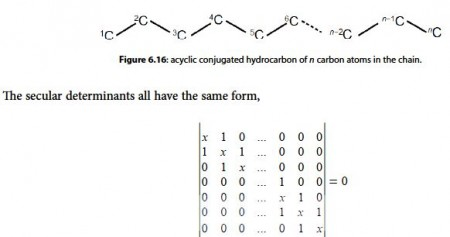for which the eigenvalues (allowed energies) are,

E = α + 2 β cos (πk / (n+1))

where n = number of carbon atoms in the conjugated chain; k = quantum number identifying the MO k = 1, 2, … n with the cosine in radians.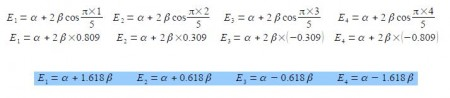These energies agree with our manual solutions found earlier. However, this generalized method becomes really useful for finding the wavefunctions (eigenvectors) for these larger Hiickel matrices. We have four different Hfickel matrices to solve, one for each energy. The coefficients are given by the following expression, note the proportionality sign as the coefficients have still to be normalized.

Cs α sin ((π k s / (n+1))

where k and n have the same meaning as before and s = atom number (1, 2, 3, … n) with the sine in radians.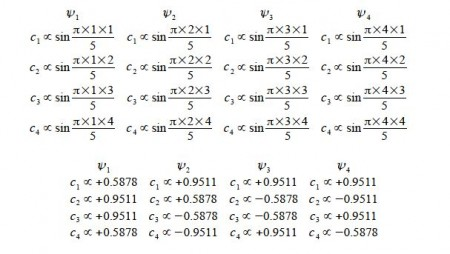Normalizing the coefficients can be done in one step for as all four wavefunctions each have two coefficients squared of 0.58782 and two of 0.95112,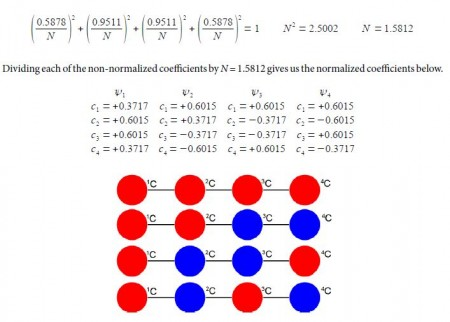Above Figure illustrates the following, firstly, ψ1 (HOMO-1) has the four n-electrons delocalized throughout the molecule. Secondly, ψ2 (HOMO) is similar to our conventional drawing of two isolated π-bonds starting at carbon atoms 1 and 3 as in the full name 1,3-butadiene. Thirdly, ψ3 (LUMO) has a single isolated double bond between atoms 2 and 3. Finally, ψ4 (LUM0+1) has four isolated 2p atomic orbitals on the carbon atoms and is completely π-antibonding. The ground electronic state has the four 2p electrons occupying ψ1 and ψ2 with spins paired.